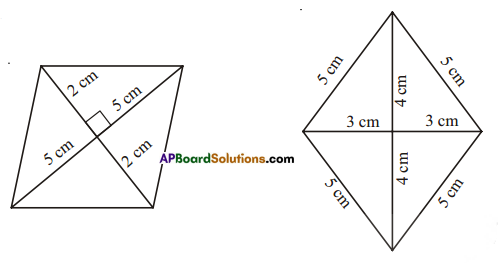# AP Board 7th Class Maths Solutions Chapter 13 Area and Perimeter Ex 4

AP State Syllabus AP Board 7th Class Maths Solutions Chapter 13 Area and Perimeter Ex 4 Textbook Questions and Answers.

## AP State Syllabus 7th Class Maths Solutions 13th Lesson Area and Perimeter Exercise 4Question 1.
Find the area of the following rhombuses.Solution:
Area = $$\frac { 1 }{ 2 }$$d1d2
d1 = 5 + 5 = 10cm
d2 = 2 + 2 = 4cm
A = $$\frac { 1 }{ 2 }$$ × 10 × 4 = 20 cm2

d1 = 3 + 3 = 6 cm
d2 = 4 + 4 = 8cm
Area = $$\frac { 1 }{ 2 }$$d1d2
= $$\frac { 1 }{ 2 }$$ × 6 × 8
= 24 cm2Question 2.
Find the missing values.

 Diagonal – 1 (d1) Diagonal – 2 (d2) Area of the rhombus 12cm 16cm 27mm 2025mm2 14m 57.6m

Solution:

 Diagonal – 1 (d1) Diagonal – 2 (d2) Area of the rhombus 12cm 16cm $$\frac{1}{2}$$ × 12 × 16 = 96cm2 27mm $$\frac{2025 \times 2}{27}$$ = 150mm 2025mm2 14m 57.6m $$\frac{1}{2}$$ × 24 × 57.6 = 691.2 m2Question 3.
If length of diagonal of a rhombus whose area 216 sq. cm. is 24 cm. when find the length of second diagonal.
Solution:
Given: Length of one diagonal d1 = 24cm, d2 =?
Area = $$\frac { 1 }{ 2 }$$d1d2 = 216
$$\frac { 1 }{ 2 }$$ × d1 × d2 = 216
$$\frac { 1 }{ 2 }$$ × 24 × d2 = 216
d2 = $$\frac{216}{12}$$ = 18Question 4.
The floor of a building consists of3 000 tiles which are rhombus shaped. The diagonals of each of the tiles are 45 cm and 30 cm. Find the total cost of polishing the floor, if cost per m2 is Rs.2.50.
Solution:
Diagonals of each (shape / rhombus) tiles
d1 = 45cm, d2 = 30cm
Total tiles = 3000
Total area = 3000 × Area of each tile = 3000 × $$\frac { 1 }{ 2 }$$ × d1 × d2
= 3000 x $$\frac { 1 }{ 2 }$$ × 45 × 30 = 2025000 cm2
= $$\frac{2025000}{100 \times 100}$$ m2 = 202.5m2
∴ Cost of polishing the floor at the rate of Rs. 2.50 per a square metre 202.5 × 2.50 = Rs. 506.25